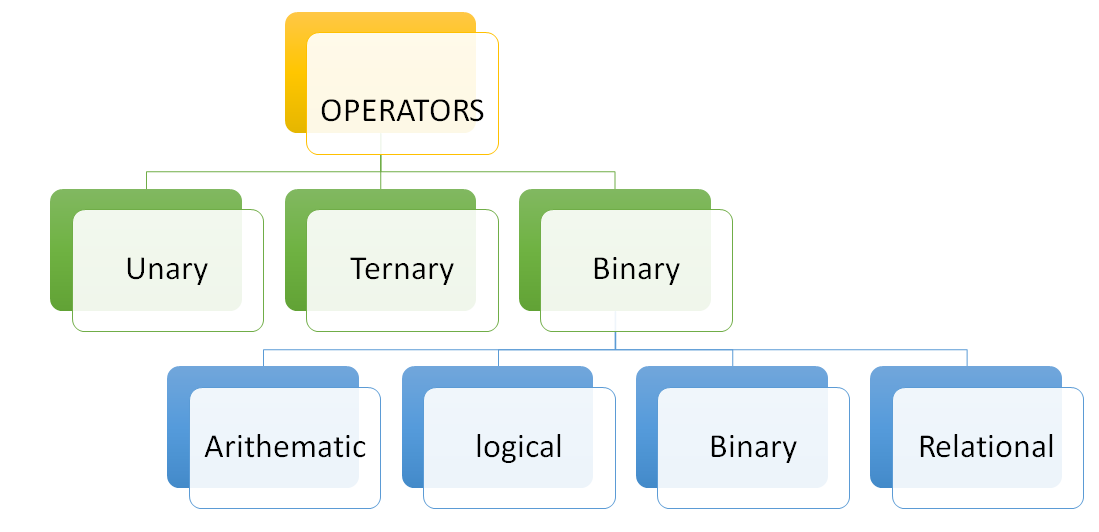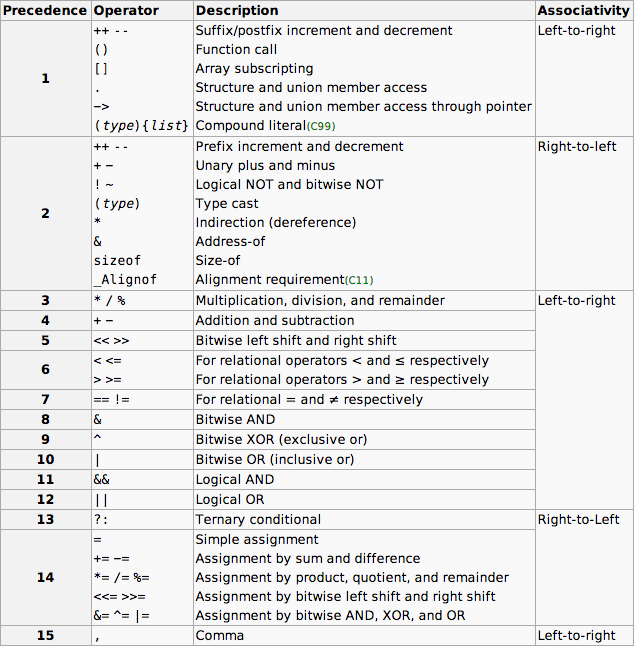# Basics of C language

In this article, we are going to learn some fundamental concepts of C Language namely Variables, Tokens, Operators in C language.
Submitted by Mansha Lamba, on August 28, 2018

Hey, Folks here I am back with my second article on C language. Hope you are through with my previous article C language - History, Popularity reasons, Characteristics, Basic structure etc. In this one, I will cover some fundamental concepts of C Language namely Variables, Tokens, Operators in C language.

Let’s get started...

### 1) Variables

They are temporary memory locations allocated during execution of the program. As the name suggests it is an entity whose value may change during program execution.

Rules for variable name

1. It does not have a space between characters.
2. Collection of alphabets, digits, and underscore (_).
3. The first character should be either alphabet or underscore (_).
4. No other special symbol except underscore (_).
5. Keywords cannot be used as variable name.

Declaration of variable

Syntax:

`datatype variable_name;`

Example:

`int a;`

It tells the user what is the data type of a declared variable or what type of values it can hold.

Initialization of a variable

The process of assigning any value to any variable.

Example:

`int a = 5;`

### 2) Token

The basic and smallest unit of C program is token.

Token includes :

1. Keywords
2. Identifier
3. Constants
4. String Constant
5. Operators
6. Special Symbols e.g: _ , @ , *

1) Keywords or Reserve words

These are those words whose meaning is already explained to the compiler.

They can’t be used as a variable name because if we do so that means we are defining the new meaning to the compiler which the compiler does not allow.

2) Identifier

They are the name given to programming elements such as array, function, variable. Same rules as of variable name.

3) Constant

Entities whose value does not during the execution of a program.

4) String constant

Collection of character enclosed by a double inverted comma. e.g.: "abc"

5) Operators

They are used to perform arithmetic and logical operations by ALU.

Example:

` a+b`

Here, a and b are operands and + is an operator.

C language has very rich operators. Many different types of operators are available in C language for different mathematical computations.

They are mainly of three types:Operators itself is a separate topic which needs to be covered in detail.

So, here we get started.

Unary operators

They require only one operand for execution, it includes :

1. Unary minus
2. Bitwise compliment
3. Logical Not
4. Increment / Decrement
5. sizeof() operator

sizeof() Operator

It is used to return the size of an operand. It can be applied on variable, constant, datatype.

Syntax:

`sizeof(operand);`

Example:

```    int a=2, b;
b = sizeof(a);
//or
b = sizeof(int);
//or
b = sizeof(5);
printf("%d\n",b);

//All of the 3 three statements will give same output.
```

Ternary operators

They are also called conditional operator. For these operators, we require 3 operands for execution. There is only and one ternary operator in C language.

Syntax:

` expression_1  ? expression_2 : expression_3 ;`

If expression_1 is true expression_2 gets executed, if false then expression_3.

Example:

```    int a=4 , b=7 , c;

c = ( a<7 ? a:b );

printf("%d\n", c );
```

Output

` 4`

Since, a = 4, exp_1 is true and therefore exp_2 gets executed. So, c = a gets executed.

Binary operators

i) Arithmetic operators

 Operator name Operator Addition + Subtraction - Multiplication * Division / Modulus %

Modulus operator gives remainder and division operator gives quotient. All arithmetic operators can be used for integer and float values except modulus which is used for integers only.

b) Relational operators

They are used for comparison between two values. They return result as true or false.

 Operator name Operator Less than < Less than or equal to <= Greater than > Greater than or equal to >= Equal to == Not equal to !=

c) Logical operators

Used to combine two relational expression and they returns result as true or false.

A B A && B A || B !A
00001
0 1 0 1 1
1 0 0 1 0
1 1 1 1 0

d) Bitwise operators

They are used to perform operation on individual bits. They can be applied on char and int.

Operators Symbol name Meaning
& Ampersand Bitwise AND
| Pipe Bitwise OR
^ Caret Bitwise X OR
~ Tilde Bitwise compliment
<< Double less than Left shift operator
>> Double greater than Right shift operator

C Operator Precedence Table:

This page lists C operators in order of precedence (highest to lowest). Their associativity indicates in what order operators of equal precedence in an expression are applied.What's New

Top Interview Coding Problems/Challenges!

Languages: » C » C++ » C++ STL » Java » Data Structure » C#.Net » Android » Kotlin » SQL
Web Technologies: » PHP » Python » JavaScript » CSS » Ajax » Node.js » Web programming/HTML
Solved programs: » C » C++ » DS » Java » C#
Aptitude que. & ans.: » C » C++ » Java » DBMS
Interview que. & ans.: » C » Embedded C » Java » SEO » HR
CS Subjects: » CS Basics » O.S. » Networks » DBMS » Embedded Systems » Cloud Computing
» Machine learning » CS Organizations » Linux » DOS
More: » Articles » Puzzles » News/Updates